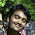## Saturday, September 19, 2009

### Assignment (Basic Proportionality Theorem and its Converse)

1. In a triangle ABC, D and E are points on the sides AB and AC respectively such that DE BC.
i) If AD=4, AE=8, DB = x-4, and EC=3x-19, find x.
ii) If AD/BD = 4/5 and EC=2.5 cm, find AE.
iii) If AD=x, DB=x-2, AE=x+2 and EC=x-1, find the value of x.
iv) If AD=2.5 cm, BD=3.0 cm and AE=3.75 cm, find the length of AC.

2. In a triangle ABC, D and E are points on the sides AB and AC respectively. For each of the following show that DE BC.
i) AB = 5.6 cm, AD = 1.4 cm, AC = 7.2 cm and AE = 1.8 cm
ii) AD = 5.7 cm, BD = 9.5 cm, AE = 3.3 cm and EC = 5.5 cm

3. In a triangle ABC, P and Q are points on sides AB and AC respectively, such that PQ BC. If AP = 2.4 cm, AQ = 2.0 cm, QC = 3.0 cm and BC = 6.0 cm, Find AB and PQ.

4. In a triangle ABC, D and E are points on AB and AC respectively such that DE BC. If AD = 2.4 cm, AE = 3.2 cm, DE = 2.0 cm and BC = 5.0 cm, find BD and CE.

5. Using basic proportionality theorem, prove that any line parallel to the parallel sides of a trapezium divides the non parallel sides proportionally.

6. In the given figure, PA, QB and RC are each perpendicular to AC.
Prove that 1/x + 1/z = 1/y.7. ABCD is a trapezium with AB CD. The diagonals AC and BD intersect each other at O. If AO = 2x+4, OC=2x-1, DO=3 and OB = 9x-21, Find x.

8. Prove that the line segments joining the mid points of adjacent sides of a quadrilateral form a parallelogram.

#### 1 comment:

1.good questions asked :)